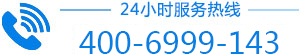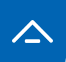• []
• []
• []
• []
• []
• []
• []
• []
• []
• []
• []
• []
• []
• []
• []
• []
• []
• []
• []
• []